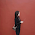# what is basic thermodynamics ?

THERMODYNAMICS : It is the field of thermal engineering that studies the properties of systems that have a temperature and involve the laws that govern the conversion of energy from one from to another, the direction in which heat will flow, and the availability of energy to do the work.

Mass and Force: Mass is one of the fundamental like time, it cannot be defined in terms of other dimensions. Much of our intuition of what mass is followed from its role in Newton;s second law of motion.

F=M*F

In this relationship, the force F required to produce a certain acceleration F of a particular body is proportional to its mass M.

Volume: The familiar property volume, is formally defined as the amount of space occupied in three-dimensional space. The SI unit of volume is cubic meters(m³).

Pressure: For a fluid system,  the pressure is defined as the normal force exerted by the fluid on a solid surface or a neighboring fluid element, per unit area. From a molecular point of view, the pressure exerted by a gas on the walls of its container is a measure of the rate at which the momentum of the molecules colliding with the wall is changed.

The SI unit for pressure is a pascal,   1Pa=1N/m³

Also commonly used unit is bar, which is defined as, 1bar=10³ Pa=10³N/m³

As a result of some practical devices measuring pressure relative to the local atmospheric pressure, we distinguish between gauge pressure and absolute pressure. Gauge pressure is defined as

Pguage =  Pabs + Patm

System: System is the fixed quantity of matter or the region that can be separated from everything else by a well-defined boundary surface. Thermodynamics system is the system on which thermodynamics investigation is done. The surface separating the system and surroundings is known as the control surface  or system boundary. The control surface may be movable or fixed. Everything beyond the system is the surroundings. A system of fixed mass is referred to as a closed system. When there is flow of mass through the control surface, the system is called an open system. An isolated system is a closed system that does not interact in any way its surroundings.

1.Yes, thermodynamics is a branch of physics that deals with heat, work, and temperature, and their relation to energy, radiation, and physical properties of matter.
precast compound wall

2.3.Thermodynamics is a branch of physics that deals with the properties of heat, work and temperature, and energy, radiation and matter. The behavior of these quantities is governed by the four laws of thermodynamics, which express a quantitative description using gross physical quantities of the mean, but can be explained in terms of microscopic components by statistical mechanics. To learn physics Try to simplify the situation as much as possible. All the concepts shared by you in this article are very important for examination. Thanks for this beneficial article.

4.A thermal store is a vessel for storing and managing heat until it is needed. It differs from a conventional hot water cylinder as the stored water is not fed to the taps directly from the thermal store. Thermal stores can provide space heating and mains pressure hot water or hot water only. MyJobQuote

Thank you for the comment